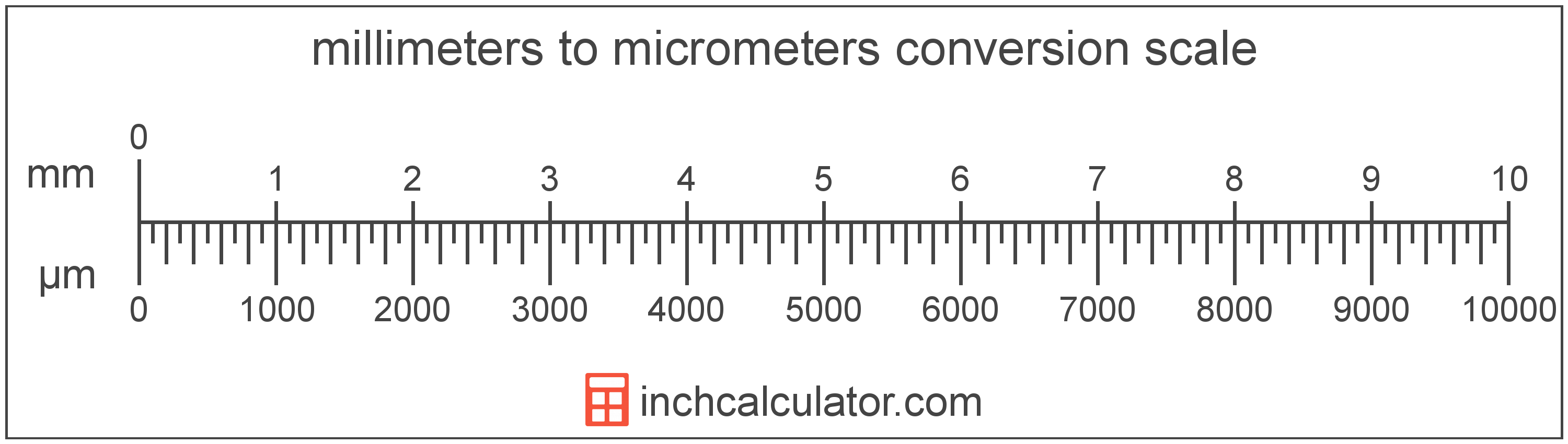# Millimeters to Micrometers Conversion

Enter the length in millimeters below to get the value converted to micrometers.

Results in Micrometers:1 mm = 1,000 µm

## How to Convert Millimeters to MicrometersTo convert a millimeter measurement to a micrometer measurement, multiply the length by the conversion ratio.

Since one millimeter is equal to 1,000 micrometers, you can use this simple formula to convert:

micrometers = millimeters × 1,000

The length in micrometers is equal to the millimeters multiplied by 1,000.

For example, here's how to convert 5 millimeters to micrometers using the formula above.
5 mm = (5 × 1,000) = 5,000 µm

### How Many Micrometers are in a Millimeter?

There are 1,000 micrometers in a millimeter, which is why we use this value in the formula above.

1 mm = 1,000 µm

Our inch fraction calculator can add millimeters and micrometers together, and it also automatically converts the results to US customary, imperial, and SI metric values.

## Millimeters

One millimeter is equal to one-thousandth (1/1,000) of a meter, which is defined as the distance light travels in a vacuum in a 1/299,792,458 second time interval.

The millimeter, or millimetre, is a multiple of the meter, which is the SI base unit for length. In the metric system, "milli" is the prefix for 10-3. Millimeters can be abbreviated as mm; for example, 1 millimeter can be written as 1 mm.

Millimeters are often represented by the smallest ticks on most metric rulers. To get a reference point of the size, the thickness of a US dime is 1.35mm.

## Micrometers

One micrometer is equal to one-millionth (1/1,000,000) of a meter, which is defined as the distance light travels in a vacuum in a 1/299,792,458 second time interval.

The micrometer, or micrometre, is a multiple of the meter, which is the SI base unit for length. In the metric system, "micro" is the prefix for 10-6. A micrometer is sometimes also referred to as a micron. Micrometers can be abbreviated as µm; for example, 1 micrometer can be written as 1 µm.

To get an idea of the actual physical length of a micrometer, one human hair is 40-50 µm thick, demonstrating how small this unit of measure is.

We recommend using a ruler or tape measure for measuring length, which can be found at a local retailer or home center. Rulers are available in imperial, metric, or combination with both values, so make sure you get the correct type for your needs.

Need a ruler? Try our free downloadable and printable rulers, which include both imperial and metric measurements.

## Millimeter to Micrometer Conversion Table

Millimeter measurements converted to micrometers
Millimeters Micrometers
0.001 mm 1 µm
0.002 mm 2 µm
0.003 mm 3 µm
0.004 mm 4 µm
0.005 mm 5 µm
0.006 mm 6 µm
0.007 mm 7 µm
0.008 mm 8 µm
0.009 mm 9 µm
0.01 mm 10 µm
0.02 mm 20 µm
0.03 mm 30 µm
0.04 mm 40 µm
0.05 mm 50 µm
0.06 mm 60 µm
0.07 mm 70 µm
0.08 mm 80 µm
0.09 mm 90 µm
0.1 mm 100 µm
0.2 mm 200 µm
0.3 mm 300 µm
0.4 mm 400 µm
0.5 mm 500 µm
0.6 mm 600 µm
0.7 mm 700 µm
0.8 mm 800 µm
0.9 mm 900 µm
1 mm 1,000 µm

## References

1. United States Mint, Coin Specifications, https://www.usmint.gov/learn/coin-and-medal-programs/coin-specifications